Mar 7, 2019 inElectromagnetic Induction Experiment Sample

## Introduction

Electricity flows in form of a current through a conductor. One of the features of a current carrying conductor is the induced magnetic field. This occurrence has been useful in the production of electric motors and appliances.  Magnetism and electricity are therefore interrelated by the fact that electric currents or the moving charges produce magnetic field that causes deflection in the magnetic compass needle.

Urgency
Pages

### Identify the scientific question in physics you chose

Some of the questions that arise in the context of electromagnetic induction are;

• Can a moving magnetic field produce electricity?
• What determines the strength of the induced current?
• Can electricity (moving current) produce magnetism?
• What determines the strength of the induced magnetism?
• What influences the type of the induced poles (N and S)?

Explain the context of your scientific question by discussing two pieces of existing research that relate to your question

To answer the above questions, the two laws discovered by veteran physicists, ampere and Faraday are useful. These include the faraday’s law of electromagnetic induction, and the Ampere’s force law.

Amperes right hand rule for coils to induce magnetic poles with the same orientation states that the opposite magnetic poles attract and the same case applies to current currying conductors . Coils will repel when the same poles are induced by current flowing through it. The discovery of Ampere’s force law of mutual action of two linear power electrical wires laid the foundation of the electrodynamics also referred to as the relationship between magnetic force acting on the general magnetic field.

In the 19th century, faraday discovered that whenever the number of magnetic lines of force in a circuit changes, an emf is induced in the circuit, referred to as the induced emf. This phenomenon is known as the electromagnetic induction.   In a closed circuit, the induced current flows through the conducting wires. The current continues t flow as long as the magnetic flux is changing.Fig1 the deflection on the galvanometer changes with the change on the magnetic flux.

A galvanometer deflects when a magnet is moved towards a stationary coil with the north pole of the magnet facing the coil, indicating the presence of the current in the circuit. The deflection is only observed when the magnet is moved towards or away from the coil. The same effect was observed when the coil was moved across a stationary magnet. It was also observed that the size of the deflection is directly proportional to the speed at which the magnet was moved. From this experiment, faraday was convinced that the effect of a moving magnet towards the coil is the same as that of the coil that is moved away from a stationary magnet.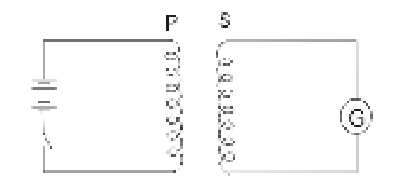Fig2 when a current is induced in the primary coil, the induced magnetic force induces current on the secondary coil causing a deflection in the needle of the galvanometer.

In fig 2, the primary coil is connected to the battery while the second coil is connected into the galvanometer.

In the above set up, the magnet in the previous set up was replaced with a current carrying coil and similar effects are observed indicating that a current carrying conductor produces magnetic field. The motion of either coil indicated a deflection in the galvanometer. The galvanometer also deflected when the switch was closed, and an opposite direction when the switch was opened.

Explain why your question is important, interesting, and relevant to you and/or to the world

The phenomenon in the set up above is useful in electric motors, electricity generation in power stations, and also in the step up and step down transformers principle of operation.

Identify the variables you will be exploring, including the following:

One experimental variable

• Number of turns on the coil

One quantitative response variable

• Voltage
• Weber

At least three controlled variables

• Length of wire
• current
• nature of the material of the wire

The magnetic `field created by moving current is directly proportional to the number of turns on the electric coil and the voltage between its terminals## Materials and Methods

List the materials and equipment needed to conduct your experiment, including all necessary measurement equipment (e.g., ruler, thermometer)

• Thin copper wire, 1m
• 2, 6 inch metal nails
• 12V lantern battery
• A variable resistor
• 1 Voltmeter
• 1 Wire cutter
• 1 Toggle switch
• 1 electric tape
• Paper clips

List the specific steps of the process used to conduct your experiment

1. The one-meter length wire was attached to one end of the positive output of the toggle switch
2. The wire was first twisted 50 times around nail 1 to create a solenoid
3. Another piece of wire was wounded 50 times around the second wire.
4. The wire was taped to the negative terminal of the variable resister whose other side was attached to the 12v battery.
5. The strength of the induced current is determined by the number of turns in the primary and secondary coil ratio, and the source voltage
6. The circuit was turned on/off and the deflection on the voltmeter was recorded.
7. The number of turns in the first nail was increased in tens and the findings were recorded in the table.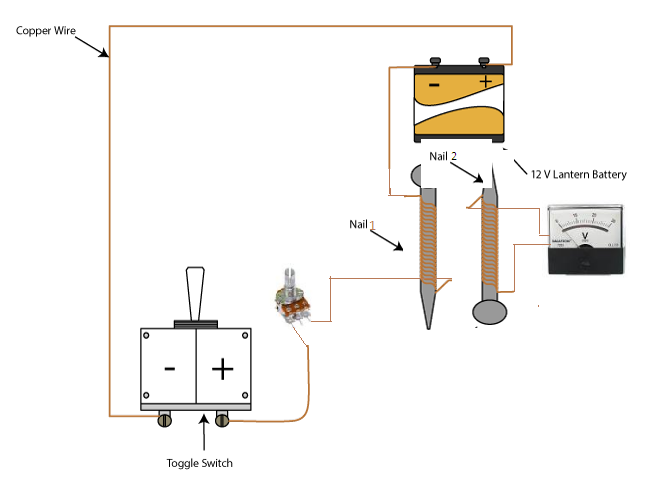Fig3 experiment set up

Explain each step in your process

• The one meter length wire was attached to one end of the positive output of the toggle switch
• This enabled the circuit to include a toggle switch which opened and closed the circuit when it was turned on/off
• The wire was first twisted 50 times around nail 1 to create a solenoid
• This created the first coil which acted as the magnet which in turn induced current to the second coil
• Another piece of wire was wounded 50 times around the second nail.
• The second nail acts as the secondary coil of the circuit
• The wire was taped to the negative terminal of the variable resister whose other side was attached to the 12v battery. The battery acts as the source of electromagnetic force which pumps the current across the circuit.
• The negative terminal of the toggle switch was connected to the positive terminal of the battery. This enabled the switch to be connected into the circuit and function properly
• The circuit was turned on/off and the deflection on the voltmeter was recorded. The turning on and off caused a magnetic flux across the primary coil which in turn induced current in the second coil
• The number of turns in the first nail was increased in tens and the findings were recorded in the table. This was done to test whether increasing the number of coils in the primary coil would affect the amount of induced current, holding the voltage constant before varying it.

Explain how you included replication in your experiment

The number of coils in the primary circuit was kept constant and the voltage was varied using a potentiometer, the readings were also recorded. This was repeated several times which led to reproduction of this same results. This way, replication was achieved in this experiment.

Explain the methods used for quantitative analysis

Readings were recorded into tables and graphs were drawn to see the relationship between the dependent and the dependent variables

Results

I. Written description

The induced voltage increased with the increase in the number of turns in the primary coil while the source voltage was maintained at 12v. The rate of turn was found to be the same when voltage was dropped to 10v.

II. Table of data collected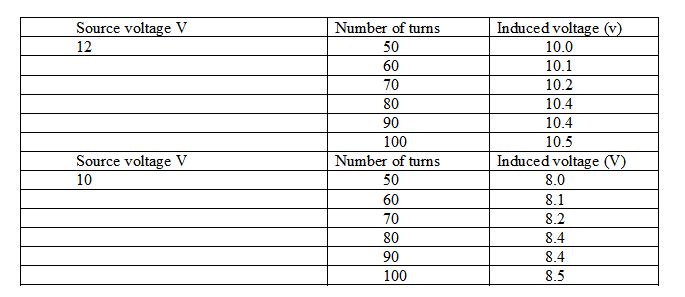III. Graph(s)

Source voltage 12v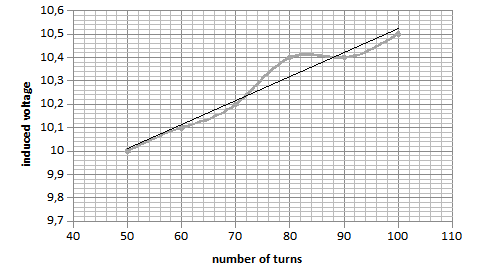Source voltage 12v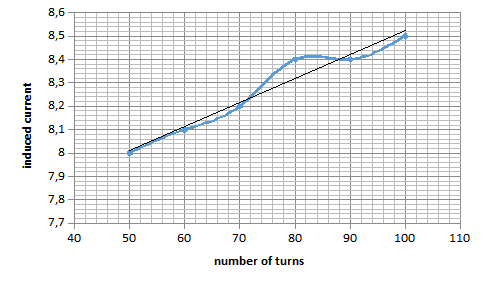Source voltage 10V

[shortcode-discount-banner]

## Conclusion

From the results above, it is evident that the magnetic `field created by moving current is directly proportional to the number of turns on the electric coil and the voltage between its terminals

Discuss how your results compare to what you expected, including any discrepancies

Electricity flows in form of current through a conductor. One of the characteristics of a current carrying conductor is the induced magnetic field. This phenomenon has been useful in the construction of electric motors and appliances.  Magnetism and electricity are therefore interrelated by the fact that electric currents or the moving charges produce magnetic field that causes deflection in the magnetic compass needle.

The observations can be summarized as

• electricity can be produced by a moving coil
• the number of turns in the primary coil and the secondary coil ratio and the source voltage determine the strength of the induced current.
• magnetism can be produced by moving current electricity (moving current) can produce magnetism
• the current flowing through the coil determines the strength of the induced magnetism.
• the type of the induced poles (N and S) is determined by the polarity of the coil terminals

The needle of the galvanometer deflects when a magnet is moved towards a stationary coil which indicates the presence of a current in the circuit. This deflection is only observed when the magnet is moved towards or away from the coil. The same effect was observed when the coil was moved across a stationary magnet. It was also observed that the size of the deflection is directly proportional to the speed at which the magnet was moved. From this experiment, faraday was convinced that the effect of a moving magnet towards the coil is the same as that of the coil that is moved away from a stationary magnet.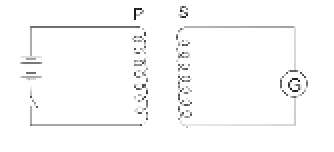Fig3 Provided that there is induction of current in the primary coil, current is induced by the induced magnetic field on the secondary coil. That results in a deflection in the galvanometer's needle

In fig 3, the primary coil is connected to the battery while the second coil is connected into the galvanometer.In the above set up, the magnet in the previous set up was replaced with a current carrying coil and similar effects are observed indicating that a current currying conductor produces magnetic field. The motion of either coil indicated a deflection in the galvanometer. The galvanometer also deflected when the switch was closed, and an opposite direction when the switch was opened. This is well demonstrated in the experiment set up.